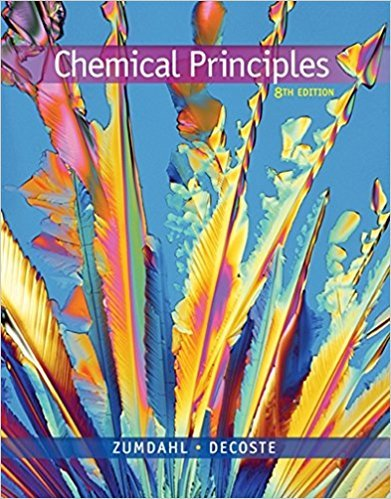×
Get Full Access to Chemical Principles - 8 Edition - Chapter 8 - Problem 8.22
Get Full Access to Chemical Principles - 8 Edition - Chapter 8 - Problem 8.22

×

# Which of the following can be classified as bufferISBN: 9781305581982 176

## Solution for problem 8.22 Chapter 8

Chemical Principles | 8th Edition

• Textbook Solutions
• 2901 Step-by-step solutions solved by professors and subject experts
• Get 24/7 help from StudySoup virtual teaching assistantsChemical Principles | 8th Edition

4 5 1 412 Reviews
26
5
Problem 8.22

Which of the following can be classified as buffer solutions? a. 0.25 M HBr 1 0.25 M HOBr b. 0.15 M HClO4 1 0.20 M RbOH c. 0.50 M HOCl 1 0.35 M KOCl d. 0.70 M KOH 1 0.70 M HONH2 e. 0.85 M H2NNH2 1 0.60 M H2NNH3NO3

Step-by-Step Solution:
Step 1 of 3

September 1, 2017 – Lecture 4 Nuclear Kinetics  dN/dt= counts per s (or min, hr, …) = slope of N vs t Rate equation dN/dt = -kN (k is rate constant) (N is number of counts) d[A]/dt = -k[A] Integrated rate equation Ln{No/N} = kt (amount of time) Ln{[Ao]/[A]} = kt (No or Ao means initial) (denominators = amount at a certain time) The Nuclear Half-Life Rates are commonly given in half-lives, the time required for one-half of a nuclide to decay Ln (No/N) = kt Ln(No/ ½ No) = kt ½ Ln(No/ ½ No) = ln2 = kt ½ Ln 2 = kt 1/2 0.693 K=ln2/ t 1/2r t1/2n2/k  Question 1: how long will a cobalt-60 source be useful if it can be used until the gamma rays it produces reach 70 percent of the original intensity 60-27 Co decays with a half-life of 5.3 years to produce 60-28

Step 2 of 3

Step 3 of 3

##### ISBN: 9781305581982

Unlock Textbook Solution